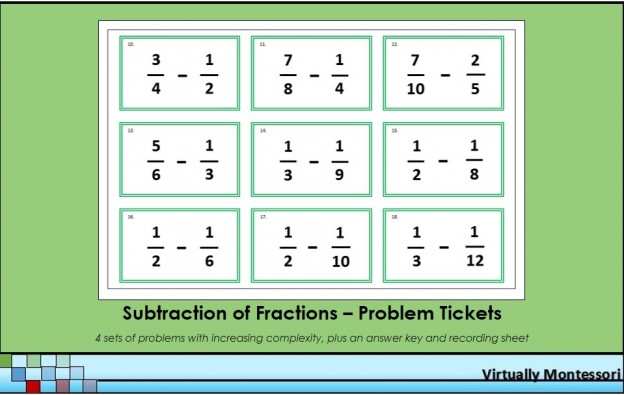# Subtraction of Fractions Problem Tickets

These fraction problems are intended to be follow-up practice after a lesson. Each group of problems adds a new layer of complexity. The green border represents the subtraction operation in Montessori math lessons and materials.

The following items are included in this material:

• Subtraction of Fractions Problem Tickets
• Problems #1-9: Subtracting simple fractions with the same denominator (can be used with Montessori metal fraction insets or fraction circles)
• Problems #10-18: Subtracting fractions with different denominators: either the minuend or subtrahend needs to be changed
• Problems #19-27: Subtracting fractions with different denominators: both the minuend and subtrahend need to be changed
• Problems #28-36: Subtracting fractions with mixed numbers: both the minuend and subtrahend need to be changed